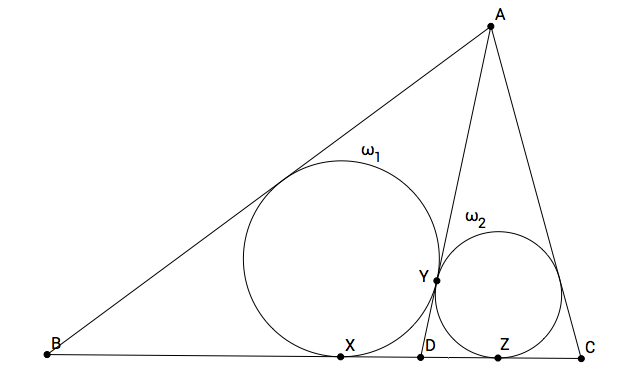# Googly Eyed Triangle

Geometry Level 4Point $D$ is on side $BC$ of $\triangle ABC.$ The incircle $\omega _1$ of $\triangle ABD$ is tangent to $BC$ at $X$ and to $AD$ at $Y.$ The incircle $\omega _2$ of $\triangle ADC$ is tangent to $BC$ at $Z$ and to $AD$ at $Y.$ If the radius of $\omega _1$ is $2,$ and the radius of $\omega _2$ is $1,$ find the value of $XY^2 + YZ^2$ to the nearest tenth.

×

Problem Loading...

Note Loading...

Set Loading...